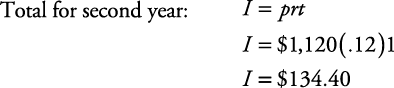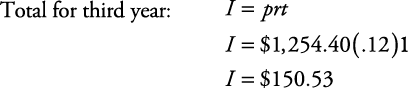## Compound Interest

Here are some examples for calculating compound interest in word problems.

##### Example 1

What will be the final total amount of money after three years on an original investment of \$1,000 if a 12% annual interest rate is compounded yearly?

First, circle what you must find— final total amount of money. Note also that interest will be compounded each year. Therefore, the solution has three parts, one for each year.Thus, the total after one year is \$1,000 + \$120 = \$1,120.Note that the principal at the beginning of the second year was \$1,120. Thus, the total after two years is \$1,120 + \$134.40 = \$1,254.40.Note that the principal at the beginning of the third year was \$1,254.40. Thus, the total after three years is \$1,254.40 + \$150.53 = \$1,404.93.